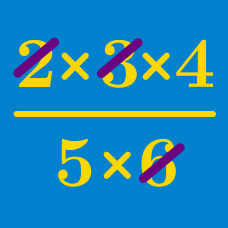Algebra

# Properties of Arithmetic Warmup

Which of these is equal to $3(\frac{5}{4} +\frac{7}{4})?$

True or False:

If you have two rational numbers, then they will always divide to produce a rational number.

You have information about how many 2-point shots, 3-point shots, and free throws (1 point) were scored by each player on a basketball team. You want to calculate how many points total were scored during the game. Which strategy will work?

A: For each player, add up the number of 2-point shots multiplied by two, 3-point shots multiplied by 3, and free throws. Then sum the totals you obtained for each player.

B: Sum the number of 3-point shots made by each player, then multiply by three. Sum the number of 2-point shots, then multiply by 2. Then add these two values to the sum of the number of free throws.

$(a-b)+c = a-(b+c)$

This statement is…

What is the value of

$(3+13+23+33+43)$ $+\text{ } (47+37+27+17+7)?$

×